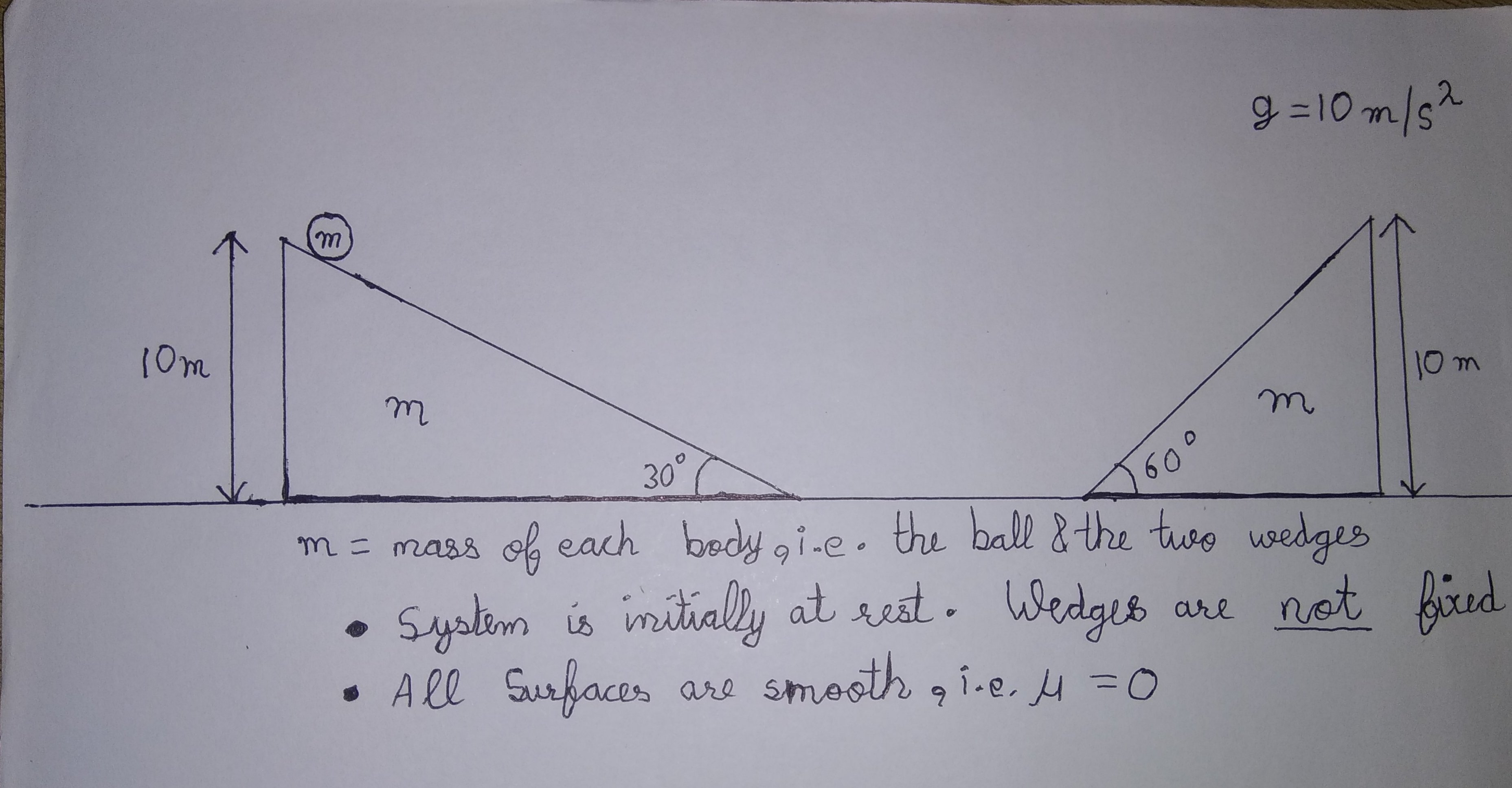# Double Wedge! (Easy Version)Find the $\color{#D61F06}{maximum}$ height reached by the ball on the $\color{#D61F06}{second}$ wedge(in m), if above system is released from rest.

# Conditions in statement

• All surfaces are $\color{#D61F06}{smooth}$.

• Mass of ball and the wedges are $\color{#D61F06}{same}$ and equal to $\color{#D61F06}{m}$

• Wedges are $\color{#D61F06}{not}$ fixed and can slide on the ground.

• Height of ball on first wedge is $\color{#D61F06}{10m}$

• Take acceleration due to gravity $\color{#D61F06}{g = 10 ms^{-2}}$

• Ball changes direction elastically each time and moves always parallel to the surface in contact.

My all time inspiration Aniket Sanghi

All of my problems are $\color{#3D99F6}{original}$

×